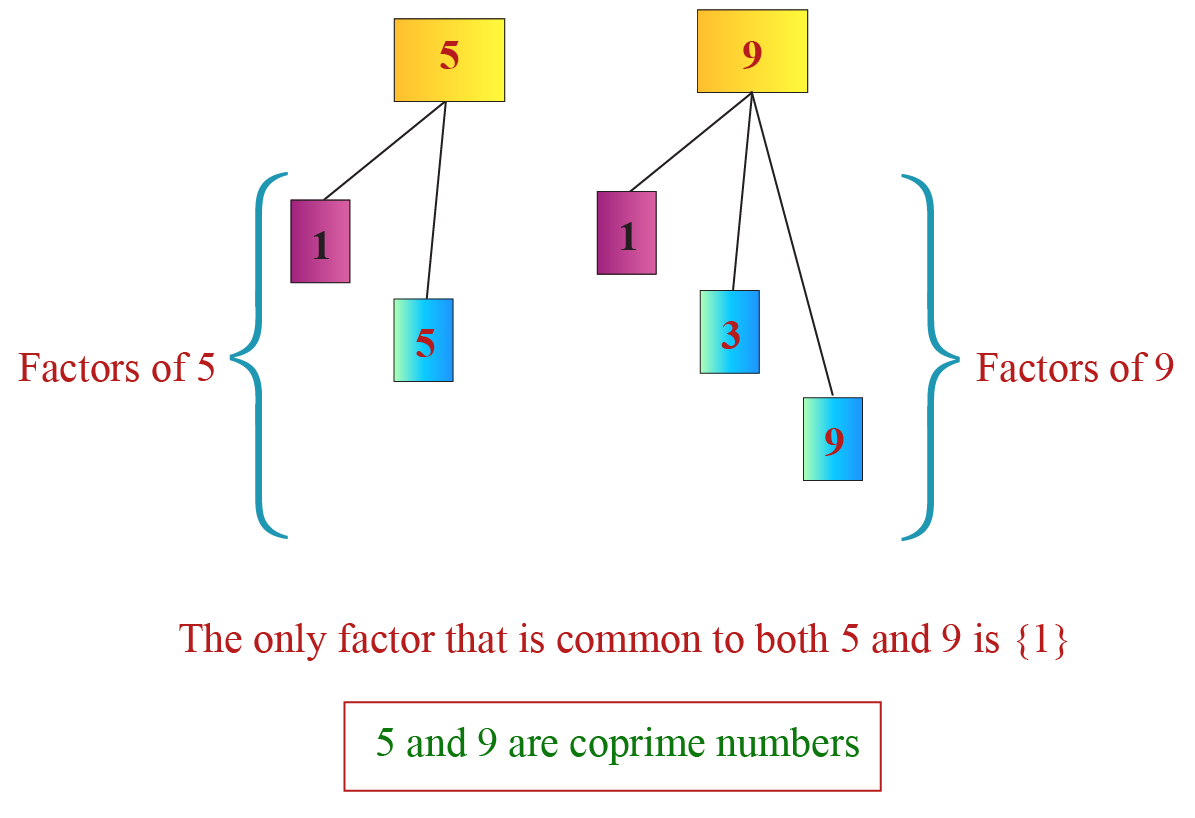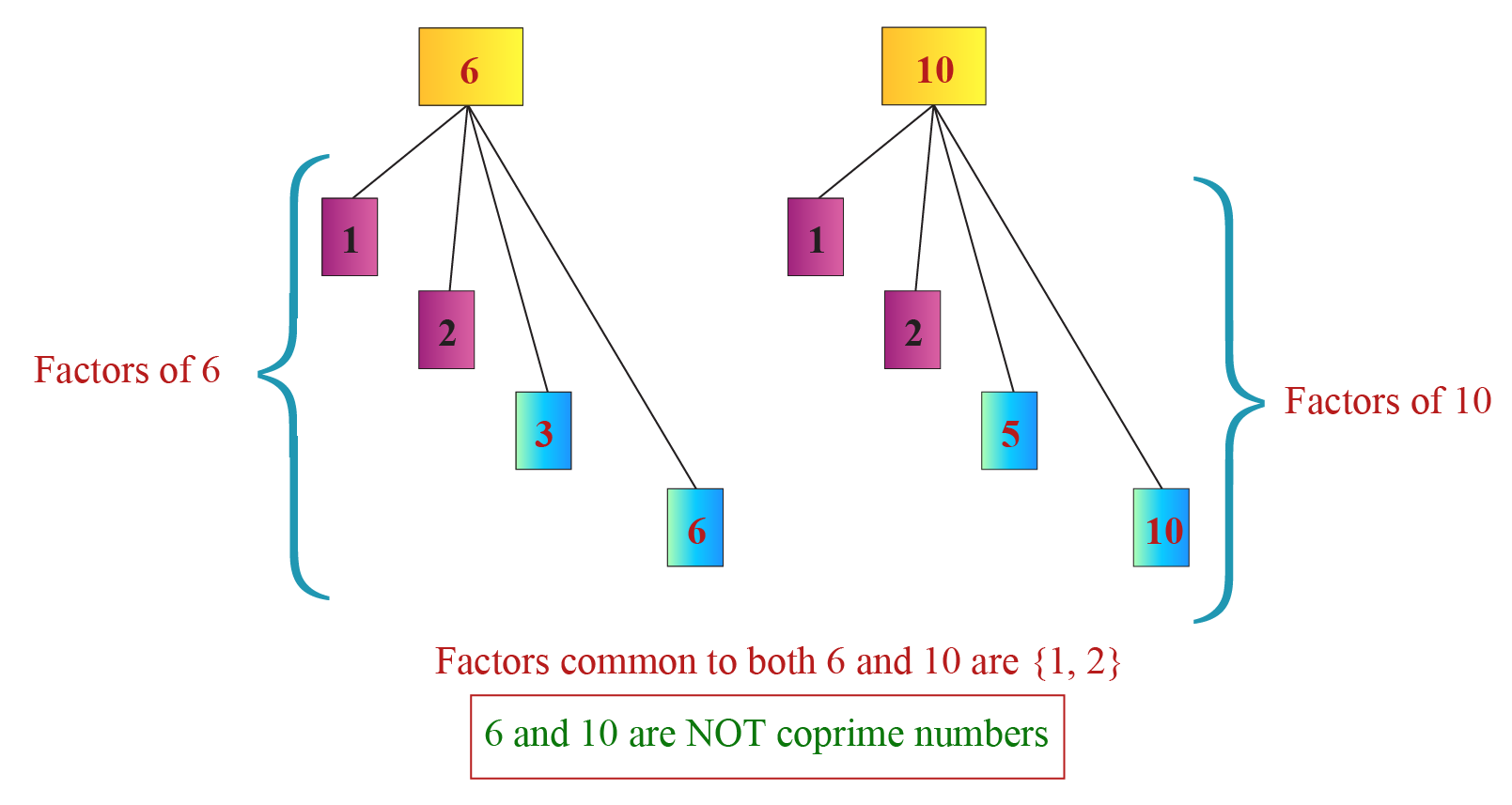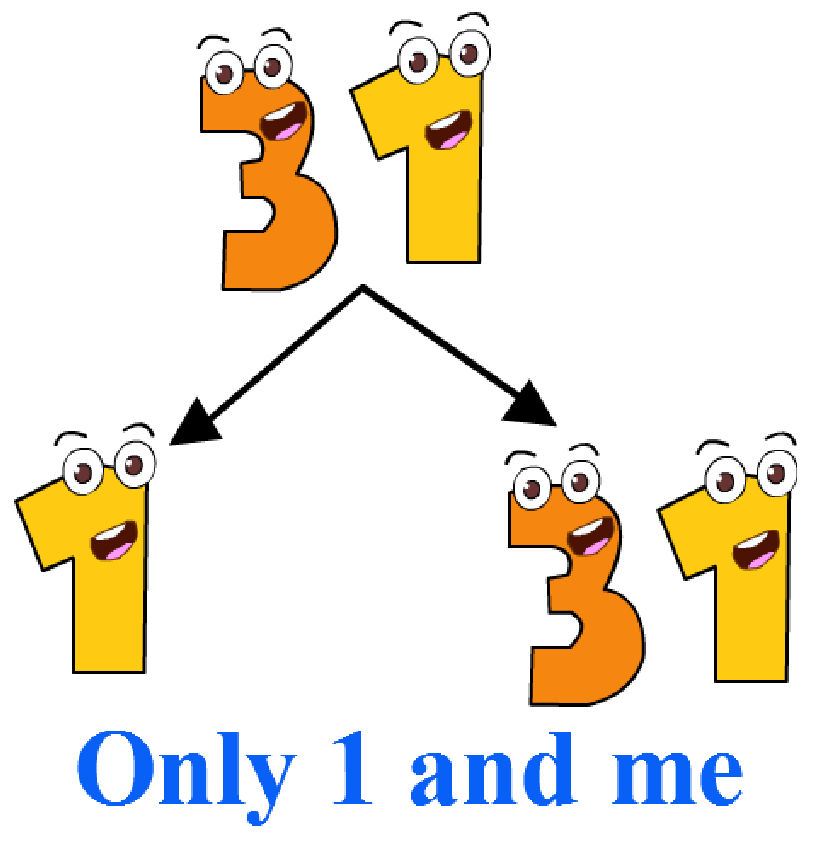# Coprime Numbers

Coprime Numbers
Go back to  'Numbers'

In this mini-lesson, we will explore the world of coprime numbers by finding the answers to the questions like how to find coprimes, how the coprimes are determined, and how to find the HCF of 2 numbers while discovering the interesting facts around them.

Two numbers are co-prime if their common factor is only 1.

In this simulation given below, enter any 2 numbers in the boxes. Click the "Get factors" button, get the factors of the numbers entered, and check if they are coprime.

Doesn't it sound interesting! Let's explore further.

## Lesson Plan

 1 What are Coprime Numbers? 2 Solved Examples on Coprime Numbers 3 Interactive Questions on Coprime Numbers 4 FREE Downloadable Resources on Coprime Numbers

## Free PDFs for Offline Revision

Get your copy of Coprime Numbers E-book along with Worksheets and Tips and Tricks PDFs for Free!

## What are Coprime Numbers?

If the only common factor of two numbers $$a$$ and $$b$$ is $$1$$, then $$a$$ and $$b$$ are coprime numbers.

In this case, $$(a, b)$$ is said to be a coprime pair.

### How to Find Coprime Numbers?

To find whether any two numbers are coprime, we first find their GCF.

If their GCF is $$1$$, we can say that they are coprime.

Example 1:

Let us consider two numbers $$5$$ and $$9$$

The factors of $$5$$ are $$1$$ and $$8$$.

The factors of $$9$$ are $$1,3$$ and $$9$$.

The factor that is common to both $$5$$ and $$9$$ is $$1$$.

GCF of $$(5,9)=1$$.

Thus, $$(5, 9)$$ is a coprime pair.Example 2:

Let us consider two numbers $$6$$ and $$10$$

The factors of $$6$$ are $$1,2,3$$ and $$6$$

The factors of $$10$$ are $$1,2,5$$ and $$10$$

Factors that are common to both $$6$$ and $$10$$ are $$1$$ and $$2$$

So GCF $$(6, 10) = 2$$

Thus, $$(6,10)$$ is NOT a coprime pair.Coprime numbers are also referred to as relatively prime or mutually prime numbers.

## Unlock the Mystical World of Coprime Numbers

Is it not fun to learn about the coprime numbers? Would you be interested in the other lessons around this concept?

 Click on a mini-lesson to explore further! Factors Composite Numbers Least Common Multiple Prime Factorization Prime Number Perfect NumbersTips and Tricks
1. Any two prime numbers are coprime.
2. Any two consecutive numbers are coprime.
3. $$1$$ forms a coprime pair with any other number.
4. A prime number is coprime with any other number that is not it's multiple.
5. Two even numbers are NEVER coprime.

## Properties of Coprime Numbers

Some properties of coprime numbers are:

• The HCF of two coprime numbers is always $$1$$

For example:

$$5$$ and $$9$$ are coprime numbers and hence, GCF $$(5, 9)=1$$

• The LCM of two coprime numbers is always their product.

For example:

$$5$$ and $$9$$ are coprime numbers.
Hence, LCM $$(5,9) = 45$$

• The sum of two coprime numbers is always coprime with their product.

For example:

$$5$$ and $$9$$ are coprime numbers.
Here, $$5+9=14$$ is coprime with $$5 \times 9=45$$

### Coprime Numbers From 1 to 100

• 2 Prime numbers have only 1 as their common factor. Consider 29 and 31.29 has 2 prime factors. 1 and 29 only31 has 2 prime factors. 1 and 31 only.

29 and 31 are prime numbers. They have only one common factor 1.

Thus they are co-prime.

We can check any two prime numbers and get them as coprime.

For example, 2 and 3, 5 and 7, 11 and 13, and so on.

• Any 2 consecutive numbers have 1 as their common factor.

Consider a few pairs of such numbers. Let us try with 14 and 15.

Factors 1,2,7,14 Common Factor Numbers 14 15 1,3,5,15

There are multiple such combinations as 1 is the only common factor.

Co-Prime Numbers- Ebook
Ebook
CoPrime Numbers -Tips and Tricks
Tips and Tricks